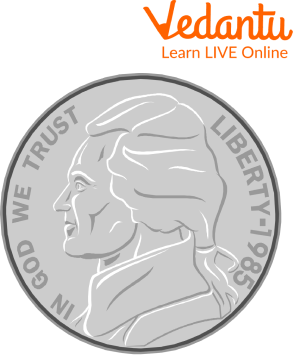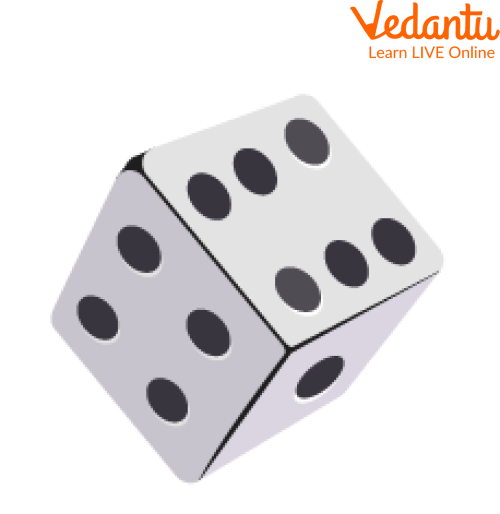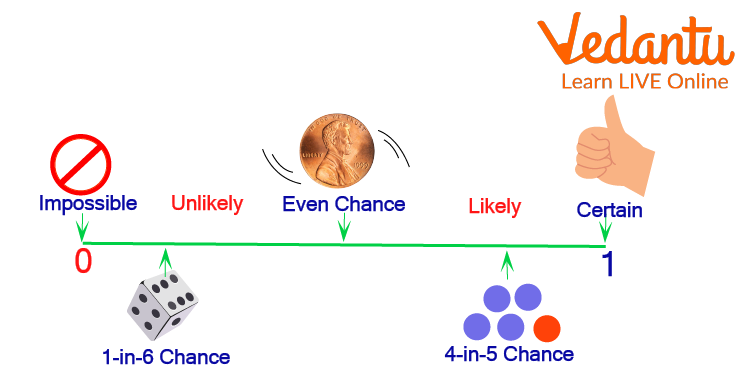Courses
Courses for Kids
Free study material
Free LIVE classes
More

# Learn About Data and ProbabilityLIVE
Join Vedantu’s FREE Mastercalss

## An Introduction to Data and Probability

You and your family were watching a match between India and Australia. Now, let’s say you're interested in knowing who won the match. You asked your father, and he informed you that team A has a chance of winning. Here, the term chance introduces the probability. There is an outcome for every event. Probability refers to these outcomes.

You then wanted to know how many wickets Team India has lost so far and the number of runs achieved by an Indian player. Your father informed you that he needed to double-check the data. So you're wondering, what exactly is data? Data is simply a collection of information. In this article, we will learn about the data and probability in detail.

## What is Data?

Data is a collection of information gathered by observations, measurements, research, or analysis. It may comprise facts, figures, numbers, names, or even general descriptions. It is a collection of facts predicted with total certainty. We can best find its chances by looking at how likely they are to happen, with the idea of probability.

## What is Probability?

Probability defines the likelihood of the occurrence of an event. There are many real-life situations in which we may have to predict the outcome of an event. We may be sure or not sure of the results of an event. In such cases, we say there is a probability of this event occurring or not occurring.

Probability is a mathematical and scientific term that describes the possibility or occurrence of an event. When a coin is tossed, for example, there is a chance that it will land on its head or tail, or we can say the probability of coming head or tail will be $\dfrac{1}{2}$.

## Probability Examples in Real Life

1. Tossing a Coin:

When a coin is tossed, there are only 2 possibilities: Head(H) or Tail(T).

So we can say that the probability of a head landing on top is $\dfrac{1}{2}$.

Also, the probability of the tail landing on top is $\dfrac{1}{2}$.Tossing a Coin

1. Throwing a Die:

When a single dice is thrown, there are 6 possible outcomes 1, 2, 3, 4, 5, and 6. Hence the probability of any number coming on top is $\dfrac{1}{6}$.Throwing a Dice

## Basic Probability Formula

In general, we can derive a theoretical formula for probability as follows:

$\text { Probability of an event occurring }=\dfrac{\text { Number of Favourable outcomes }}{\text { Total number of outcomes }}$

Also, the experimental probability is found when an experiment is performed.

$P(\text { Event })=\dfrac{\text { Total number of favourable outcomes of an event }}{\text { Total number of times the experiment is made }}$

For example, if you toss a coin 10 times, and heads occur 4 times, then

$P(Head)=\dfrac{4}{10}$

$P(Tail)=\dfrac{6}{10}$Probability

For example: Suppose there is a probability activity in which we have a bag with 5 red and 2 blue balls. Find the probability of taking out a red ball.

Here the total number of balls is 5+2=7

The number of red balls is 5.

As we know that the, $\text { Probability of an event occurring }=\dfrac{\text { Number of Favourable outcomes }}{\text { Total number of outcomes }}$

Therefore, on substituting the values, we get

P(Red ball) =$\dfrac{5}{7}$

Hence the probability of getting a red ball is $\dfrac{5}{7}$.

## Solved Examples

Q 1. Find the probability of chances of rolling a “2” with a die.

Ans: The number of ways a dice will roll “2” is ‘1’.

Since there is only 1 face with ‘2’ on it.

Hence, the total number of outcomes = 6

As we know that the, $\text { Probability of an event occurring }=\dfrac{\text { Number of favourable outcomes }}{\text { Total number of outcomes }}$

A dice has 6 faces.

Therefore, on substituting the values, we get

P(Rolling a 2)= $\dfrac{1}{6}$

Hence by formula, its probability will be $\dfrac{1}{6}$

Q 2. A dice is thrown. What is the probability of getting an even number?

Ans: When a dice is thrown, the total number of outcomes is 6.

Now, chance of getting an even number = (2,4,6) = 3

Hence the probability of getting an even number is $\dfrac{3}{6}$, and that is $\dfrac{1}{2}$.

## Practice Questions

Q 1. There are 6 candies in a jar. 3 are blue, 2 are red, and 1 is yellow. What is the probability of picking red candy? (Ans: $\dfrac{1}{3}$)

Q 2. Suppose there are 12 marbles in a bag, 7 are red, and 5 are blue. What is the probability of red marble? (Ans: $\dfrac{7}{12}$)

## Summary

In this article, we have seen what probability and data are. As we have seen, probability is just the chance of something occurring. When we are uncertain about the result of an event, we might discuss the probabilities of several outcomes—how likely they are. At the same time, data is the collection of information. For example, we need data like a coin or a die to make certain things happen. Later on, we saw some solved examples and practice problems. After going through the article, make sure you give it a try to practise the problem, as it will help you to understand the topic much better.

Last updated date: 25th Sep 2023
Total views: 84.6k
Views today: 0.84k

## FAQs on Learn About Data and Probability

1. What type of data can come under probability?

A probability distribution is a statistical function describing all possible values and likelihoods a random variable can take within a given range.

2. What are the uses of probability?

Probability provides information about the likelihood that something will happen. For instance, Meteorologists use weather patterns to predict the probability of rain. In epidemiology, we use probability theory to understand the relationship between exposures and the risk of health effects.

3. Is probability used in data science?

Probability and Statistics form the basis of Data Science. Predictions and estimates form a crucial part of Data Science. The probability theory helps predict the prediction. Statistical methods make estimates.